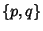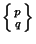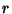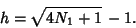## Quasiregular Polyhedron

A quasiregular polyhedron is the solid region interior to two Dual regular polyhedra with Schläfli Symbolsand. Quasiregular polyhedra are denoted using a Schläfli Symbol of the form, with(1)

Quasiregular polyhedra have two kinds of regular faces with each entirely surrounded by faces of the other kind, equal sides, and equal dihedral angles. They must satisfy the Diophantine inequality(2)

But, somust be 2. This means that the possible quasiregular polyhedra have symbols,, and. Now(3)

is the Octahedron, which is a regular Platonic Solid and not considered quasiregular. This leaves only two convex quasiregular polyhedra: the Cuboctahedronand the Icosidodecahedron.

If nonconvex polyhedra are allowed, then additional quasiregular polyhedra are the Great Dodecahedronand the Great Icosidodecahedron(Hart).

For faces to be equatorial,(4)

The Edges of quasiregular polyhedra form a system of Great Circles: the Octahedron forms three Squares, the Cuboctahedron four Hexagons, and the Icosidodecahedron six Decagons. The Vertex Figures of quasiregular polyhedra are Rhombuses (Hart). The Edges are also all equivalent, a property shared only with the completely regular Platonic Solids.

Coxeter, H. S. M. Quasi-Regular Polyhedra.'' §2-3 in Regular Polytopes, 3rd ed. New York: Dover, pp. 17-20, 1973.
Hart, G. W. Quasi-Regular Polyhedra.'' http://www.li.net/~george/virtual-polyhedra/quasi-regular-info.html.